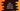# Dart HashSet where and whereType explanation with examples## How to use where and whereType in Dart HashSet:

The where and whereType methods of Dart HashSet are used to get an iterable of elements satisfied by a given function. In this post, we will learn how to use where and whereType with examples.

### How to use the where method:

The syntax of the where method is:

``where(bool fn(E e)) → Iterable<E>``
• It takes one function as its parameter and returns one iterable.
• The function must return a boolean value. If the return value for any element is true, it will add that element to the iterable. Otherwise, it will skip that element.

Let’s take one example to understand how this method works:

``````import 'dart:collection';

void main() {
final hashSet = HashSet.of([1, 2, 3, 4, 5, 6, 7, 8, 9]);
final evenIterable = hashSet.where((e) => e.isEven);

print('Original HashSet: \${hashSet}');

print('Content of the evenIterable: ');
evenIterable.forEach((e) {
print(e);
});
}``````
• The hashSet is a HashSet of numbers.
• It uses the where method to find the even numbers of the hashSet and assigns the returned iterable to the evenIterable variable.
• The last two print statements are printing the content of the original HashSet and the content of the iterable.

It will give the below result:

``````Original HashSet: {1, 2, 3, 4, 5, 6, 7, 8, 9}
Content of the evenIterable:
2
4
6
8``````

As you can see that the even numbers are picked from the given HashSet.

### How to use the whereType method:

The whereType method is used to pick elements of a specific type. For example, if a HashSet contains both string and integer values, we can pick only the string or integer values by using whereType.

The syntax of the whereType method is:

``whereType<T>() → Iterable<T>``

Where T is the type. It returns one lazy iterable.

The below example program shows how to use the whereType method:

``````import 'dart:collection';

void main() {
final hashSet = HashSet.of(['a', 1, 'b', 2, 'c', 3, 'd', 4, 'e', 5]);
final stringSetIterable = hashSet.whereType<String>();
final integerSetIterable = hashSet.whereType<int>();

print('Original HashSet: \${hashSet}');

print('String values of the HashSet: \${stringSetIterable}');
print('Integer values of the HashSet: \${integerSetIterable}');
}``````

Here,

• The HashSet holds both string and integer variables.
• We are using the whereType method to filter out string and integer values of the HashSet. The string values are assigned to the stringSetIterable and the integer values are assigned to the integerSetIterable variable.

If you run this program, it will print:

``````Original HashSet: {e, 1, a, 2, 3, 4, 5, d, c, b}
String values of the HashSet: (e, a, d, c, b)
Integer values of the HashSet: (1, 2, 3, 4, 5)``````

As you can see, the strings and integer values of the HashSet are picked by the whereType method.